# Hölder inequality

(diff) ← Older revision | Latest revision (diff) | Newer revision → (diff)

The Hölder inequality for sums. Letand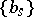be certain sets of complex numbers,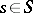, whereis a finite or an infinite set of indices. The following inequality of Hölder is valid:(1)

where,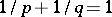; this inequality becomes an equality if and only if, and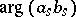andare independent of. In the limit case, when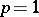,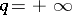, Hölder's inequality has the form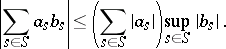If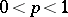, Hölder's inequality is reversed. The converse proposition of Hölder's inequality for sums is also true (M. Riesz): Iffor all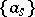such that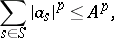then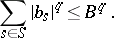For sums of a more general form, Hölder's inequality takes the form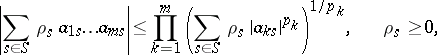if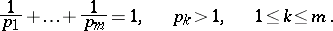(2)

The Hölder inequality for integrals. Letbe a Lebesgue-measurable set in an-dimensional Euclidean spaceand let the functions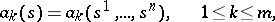belong to, condition

being satisfied. The following inequality of Hölder is then valid: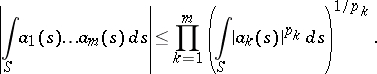If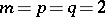, one obtains the Bunyakovskii inequality. Analogous remarks (concerning the sign and the limit case) as were made for the Hölder inequality

are also valid for the Hölder inequality for integrals.

In the Hölder inequality the setmay be any set with an additive function(e.g. a measure) specified on some algebra of its subsets, while the functions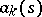,, are-measurable and-integrable to degree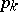.

The generalized Hölder inequality. Letbe an arbitrary set, let a (finite or infinite) functional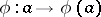be defined on the totality of all positive functions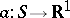and let this functional satisfy the following conditions: a)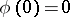; b)for all numbers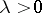; c) if, then the inequality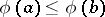is valid; and d)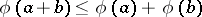. If the conditions

are also met, the generalized Hölder inequality is valid for the functional:How to Cite This Entry:
Hölder inequality. Encyclopedia of Mathematics. URL: http://encyclopediaofmath.org/index.php?title=H%C3%B6lder_inequality&oldid=14455
This article was adapted from an original article by L.P. Kuptsov (originator), which appeared in Encyclopedia of Mathematics - ISBN 1402006098. See original article